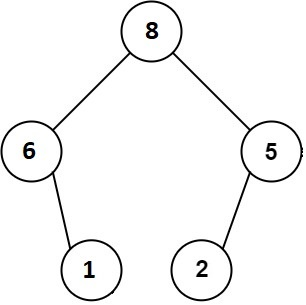# Maximum Binary Tree

Posted: 22 Mar, 2021
Difficulty: Moderate

## PROBLEM STATEMENT

#### You have been given an array/list ‘TREE’ having ‘N’ unique integers. You need to make a maximum binary tree recursively from ‘TREE’ using the following conditions:

``````1. Create a root of the maximum binary tree whose value is the maximum value present in the ‘TREE’.
2. Recursively build the left subtree on the subarray prefix to the left of the maximum value.
3. Recursively build the right subtree on the subarray suffix to the right of the maximum value.
``````

#### For example:

``````For ‘TREE’ = [6, 1, 8, 2, 5],see the maximum binary tree in the below picture for reference:
````````````As we can see the root of the maximum binary tree as shown in the picture is ‘8’ which is the maximum value in the ‘TREE’. The left subtree contains all the values which are present in the left of 8  in ‘TREE’ and the right subtree contains all the values which are present in the right of ‘8’ in ‘TREE’. Similarly, the maximum value in the left subarray of 8 is 6 so 6 becomes the root of the left subtree, 5 is the maximum value in the right subarray of the 8 so 5 becomes the root of the right subarray, and so on.
``````
##### Input Format
``````The first line of input contains an integer 'T' representing the number of test cases. Then the test cases follow.

The first line of each test case contains an integer ‘N’ representing the number of elements in the ‘TREE’.

The second line of each test case contains ‘N’ space separated integers denoting the values of ‘TREE’.
``````
##### Output Format :
``````For each test case, print the level order traversal of the maximum binary tree formed using the array/list ‘TREE’.

The output for each test case is printed in a separate line.
``````
##### Note:
``````You are not required to print the expected output; it has already been taken care of, Just implement the function.
``````
##### Constraints :
``````1 <= T <= 100
1 <= N <= 10 ^ 4
1 <= TREE[i] <= 10 ^ 4

Time limit: 1 second
``````Approach 1

This approach aims to find the maximum element in ‘TREE’ and make it the root of the current binary tree. For the left and right subtree, we use the same approach using the left and right subarray, respectively.

We use the function ‘CONSTRUCT_MAX_TREE’ with three arguments ‘TREE’, ‘l’ and ‘r’, which will return the root of the maximum binary tree consisting of numbers within the indices ‘l’ and ‘r’ of the ‘TREE’.

Here is the complete algorithm:

• We call the function ‘CONSTRUCT_MAX_TREE’  and pass ‘TREE’, 0 and ‘N’.
• Find the index of the maximum element in the range ‘l’ to ‘r’ and store it in ‘MAX_ELEMENT_INDEX’.
• Make the element at ‘MAX_ELEMENT_INDEX’ index as the local root node.
• Determine the left child of the root using the function ‘CONSTRUCT_MAX_TREE’ and pass ‘TREE’, ‘l’, ‘MAX_ELEMENT_INDEX’. Doing this recursively finds the largest element in the subarray left to the current largest element.
• Determine the right child of the root using the function ‘CONSTRUCT_MAX_TREE’ and pass ‘TREE’, ‘MAX_ELEMENT_INDEX + 1’, ‘r’. Doing this recursively finds the largest element in the subarray right to the current largest element.
• Return the root node to the calling function.